The PHREG Procedure
 CONTRAST Statement
CONTRAST ’label’ row-description <,...row-description></options> ;

where a row-description is: effect values <,...effect values>

The CONTRAST statement provides a mechanism for obtaining customized hypothesis tests. It is similar to the CONTRAST statement in PROC GLM and PROC CATMOD, depending on the coding schemes used with any categorical variables involved.

The CONTRAST statement enables you to specify a matrix,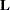, for testing the hypothesis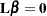. You must be familiar with the details of the model parameterization that PROC PHREG uses (for more information, see the PARAM= option in the section CLASS Statement). Optionally, the CONTRAST statement enables you to estimate each row,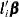, of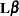and test the hypothesis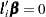. Computed statistics are based on the asymptotic chi-square distribution of the Wald statistic.

There is no limit to the number of CONTRAST statements that you can specify, but they must appear after the MODEL statement.

The following parameters are specified in the CONTRAST statement:

label

identifies the contrast on the output. A label is required for every contrast specified, and it must be enclosed in quotes.

effect

identifies an effect that appears in the MODEL statement. You do not need to include all effects that are included in the MODEL statement.

values

are constants that are elements of thematrix associated with the effect. To correctly specify your contrast, it is crucial to know the ordering of parameters within each effect and the variable levels associated with any parameter. The "Class Level Information" table shows the ordering of levels within variables. The E option, described later in this section, enables you to verify the proper correspondence of values to parameters.

The rows ofare specified in order and are separated by commas. Multiple degree-of-freedom hypotheses can be tested by specifying multiple row-descriptions. For any of the full-rank parameterizations, if an effect is not specified in the CONTRAST statement, all of its coefficients in thematrix are set to 0. If too many values are specified for an effect, the extra ones are ignored. If too few values are specified, the remaining ones are set to 0.

When you use effect coding (by specifying PARAM=EFFECT in the CLASS statement), all parameters are directly estimable (involve no other parameters). For example, suppose an effect coded CLASS variable A has four levels. Then there are three parameters (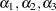) representing the first three levels, and the fourth parameter is represented by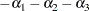To test the first versus the fourth level of A, you would test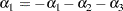or, equivalently,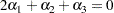which, in the form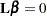, is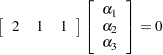Therefore, you would use the following CONTRAST statement:

```contrast '1 vs. 4' A 2 1 1;
```

To contrast the third level with the average of the first two levels, you would test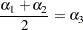or, equivalently,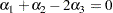Therefore, you would use the following CONTRAST statement:

```contrast '1&2 vs. 3' A 1 1 -2;
```

Other CONTRAST statements involving classification variables with PARAM=EFFECT are constructed similarly. For example:

```contrast '1 vs. 2    '  A  1 -1  0;
contrast '1&2 vs. 4  '  A  3  3  2;
contrast '1&2 vs. 3&4'  A  2  2  0;
contrast 'Main Effect'  A  1  0  0,
A  0  1  0,
A  0  0  1;
```

When you use the less-than-full-rank parameterization (by specifying PARAM=GLM in the CLASS statement), each row is checked for estimability. If PROC PHREG finds a contrast to be nonestimable, it displays missing values in corresponding rows in the results. PROC PHREG handles missing level combinations of categorical variables in the same manner as PROC GLM. Parameters corresponding to missing level combinations are not included in the model. This convention can affect the way in which you specify thematrix in your CONTRAST statement. If the elements ofare not specified for an effect that contains a specified effect, then the elements of the specified effect are distributed over the levels of the higher-order effect just as the GLM procedure does for its CONTRAST and ESTIMATE statements. For example, suppose that the model contains effects A and B and their interaction A*B. If you specify a CONTRAST statement involving A alone, thematrix contains nonzero terms for both A and A*B, since A*B contains A.

The Cox model contains no explicit intercept parameter, so it is not valid to specify one in the CONTRAST statement. As a consequence, you can test or estimate only homogeneous linear combinations (those with zero-intercept coefficients, such as contrasts that represent group differences) for the GLM parameterization.

The degrees of freedom are the number of linearly independent constraints implied by the CONTRAST statement—that is, the rank of.

You can specify the following options after a slash (/).

ALPHA= p

specifies the level of significancefor the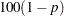% confidence interval for each contrast when the ESTIMATE option is specified. The valuemust be between 0 and 1. By default,is equal to the value of the ALPHA= option in the PROC PHREG statement, or 0.05 if that option is not specified.

E

requests that thematrix be displayed.

ESTIMATE=keyword

requests that each individual contrast (that is, each row,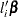, of) or exponentiated contrast (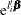) be estimated and tested. PROC PHREG displays the point estimate, its standard error, a Wald confidence interval, and a Wald chi-square test for each contrast. The significance level of the confidence interval is controlled by the ALPHA= option. You can estimate the contrast or the exponentiated contrast (), or both, by specifying one of the following keywords:

PARM

specifies that the contrast itself be estimated.

EXP

specifies that the exponentiated contrast be estimated.

BOTH

specifies that both the contrast and the exponentiated contrast be estimated.

SINGULAR=number

tunes the estimability check. This option is ignored when the full-rank parameterization is used. Ifis a vector, define ABS() to be the largest absolute value of the elements of. For a row vector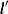of the contrast matrix, defineto be equal to ABS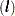if ABSis greater than 0; otherwise,equals 1. If ABS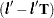is greater than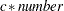, thenis declared nonestimable. The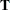matrix is the Hermite form matrix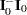, where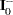represents a generalized inverse of the information matrix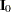of the null model. The value for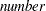must be between 0 and 1; the default value is 1E4.

TEST<(keywords)>
requests a Type 3 test for each contrast. The default is to use the Wald statistic, but you can requests other statistics by specifying one or more of the following keywords:
ALL

requests the likelihood ratio tests, the score tests, and the Wald tests. Specifying TEST(ALL) is equivalent to specifying TEST=(LR SCORE WALD).

NONE

suppresses the Type 3 analysis. Even if the TEST option is not specified, PROC PHREG displays the Wald test results for each model effect if a CLASS variable is involved in a MODEL effect. The NONE option can be used to suppress such display.

LR

requests the likelihood ratio tests. This request is not honored if the COVS option is also specified.

SCORE

requests the score tests. This request is not honored if the COVS option is also specified.

WALD

requests the Wald tests.Previous Page | Next Page | Top of Page